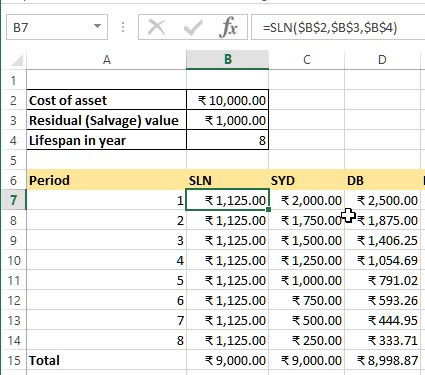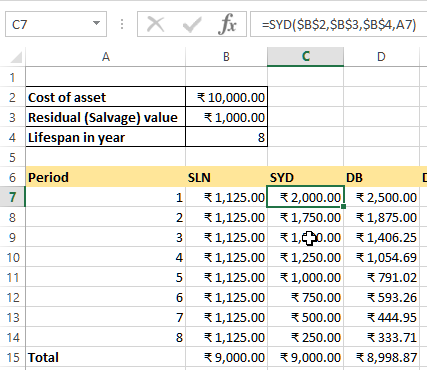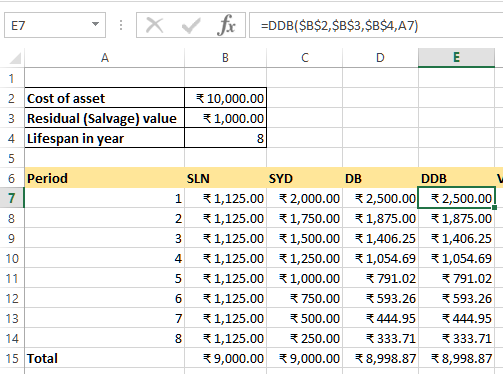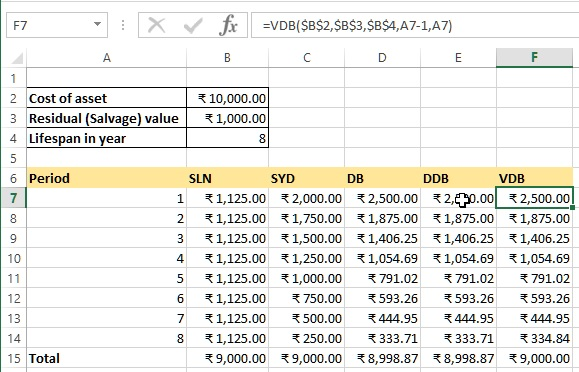# Depreciation calculations in Excel

##### How to calculate depreciation of an asset in Excel?

In last post, we learnt about how to calculate compounded interest in MS Excel. In this post, we shall learn how to calculate different types of depreciation on assets costs in Excel.

Excel has got 5 different methods to calculate depreciation. Let's learn them one by one.

### SLN function

SLN (Straight Line) function is used to return the straight-line depreciation of an assets for one period. In B7 cell, we have '=SLN(\$B\$2,\$B\$3,\$B\$4)' formula. Notice that we used Absolute cell reference in the formula so that when we copy-paste it to other relevant cells, the cell reference get sticked. Here are the arguments of SLN function

• \$B\$2 is the cost of an asset
• \$B\$3 is the salvage value (we also called Salvage value) of the asset
• \$B\$4 is the total expected life of the assetWhen we drag the same formula to other B7 to B14 cells, we get following result. When we sum the values of B7 to B14, we get 9,000 (The asset value - residual value) as shown in B15 cell.### SYD function

SYD (Sum of Years's Digits) function in Excel returns the sum-of-years' digits depreciation of an asset for a specific period. In C7 cell, we have '=SYD(\$B\$2,\$B\$3,\$B\$4,A7)' formula that accepts

• 1st parameter is Cost
• 2nd parameter is Salvage value
• 3rd parameter is Life
• 4th parameter is Period
Dragging the same formula till C14, we get depreciation each year as shown in the picture below.### DB function

DB (Declining Balance) function is used to return the depreciation of an assets for a specific period using fixed-decline balance method. In D7 cell, we have '=DB(\$B\$2,\$B\$3,\$B\$4,A7)' formulat that accepts

• 1st parameter is Cost
• 2nd parameter is Salvage value
• 3rd parameter is Life
• 4th parameter is Period
these are same parameter as SYD function and the result is below.### DDB function

DDB (Double Declining Balance) function is used to return the depreciation of an assets for a specific period using double-declining balance method. In E7 cell, we have '=DDB(\$B\$2,\$B\$3,\$B\$4,A7)' that accepts

• 1st parameter is Cost
• 2nd parameter is Salvage value
• 3rd parameter is Life
• 4th parameter is Period
same as SYD and DB functions. The result is below.### VDB function

VDB (Variable Declaring Balance) function is similar as DDB function however we can specify period (starting and ending) as 4th and 5th parameters. F7 cell has '=VDB(\$B\$2,\$B\$3,\$B\$4,A7-1,A7)' formula that accepts following parameters

• 1st parameter is Cost
• 2nd parameter is Salvage value
• 3rd parameter is Life
• 4th parameter is Start period
• 5th parameter is End Period

the result is displayed below.You may notice that there is slight difference in total for DDB and VDB values, this is because VDB function is intelligent enough to switch to Straight Line calculation when it sees that the last period is not reaching to the (Cost - Salvage amount).

Hope you liked this tutorials post. Do share to your friends and colleagues !

Views: 4303 | Post Order: 36